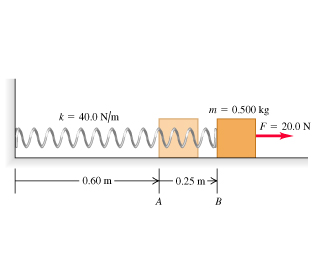# Problem: A 0.500-kg block, attached to a spring with length 0.60 m and force constant 40.0 N/m, is at rest with the back of the block at point exttip{A}{A} on a frictionless, horizontal air table (the figure ). The mass of the spring is negligible. You move the block to the right along the surface by pulling with a constant 20.0-N horizontal force.What is the blocks speed when the back of the block reaches point exttip{B}{B}, which is 0.25 m to the right of point exttip{A}{A}?When the back of the block reaches point exttip{B}{B}, you let go of the block. In the subsequent motion, how close does the block get to the wall where the left end of the spring is attached?

⚠️Our tutors found the solution shown to be helpful for the problem you're searching for. We don't have the exact solution yet.

###### Problem Details

A 0.500-kg block, attached to a spring with length 0.60 m and force constant 40.0 N/m, is at rest with the back of the block at point on a frictionless, horizontal air table (the figure). The mass of the spring is negligible. You move the block to the right along the surface by pulling with a constant 20.0-N horizontal force.

What is the blocks speed when the back of the block reaches point , which is 0.25 m to the right of point ?

When the back of the block reaches point , you let go of the block. In the subsequent motion, how close does the block get to the wall where the left end of the spring is attached?